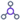Configure a Formula on an eForm

To configure a formula on an eForm, do the procedure in this topic.

How to Start

1. Open eForm Builder.

For information about how to open this screen, refer to eForm Builder screen.

2. On the Advanced Controls tab, drag a Formulaform control onto your eForm.
3. Complete the fields as necessary.
4. On the eForm Builder screen, click the Formula form control, and click Formula.

Procedure

1. On the Formula Builder screen, in the Fields/Tokens list, select the form control to create a formula.
2. In the Operators list, select the operator to compare the values in your logical expression.
Field Name Definition

Operators

Function:
Specifies the operator to use in the formula.
Accepted Values:
• +
• -
• *
• /
• %
• <=
• <
• >=
• >
• !=
• =
• &&
• ||
Default Value:
None
Example:
Refer to:
3. (Optional) In the Keywords field, select the keyword to use in your formula.
Field Name Definition

Keywords

Function:
Shows the list of keywords to use in the formula to show specific values on the eForm.
Accepted Values:
• randomstring - Let's you use a random alphanumeric string on the eForm which can be used to validate the form user.
• now - Let's you use the current day in the formula.
• days - Let's you use the current day as a number. For example, Sunday is 0, Monday is 1 and so on.
• months - Let's you use the month in the formula.
• years- Let's you use the year in the formula.
Default Value:
• randomstring
• now
• days
• months
• years
4. In the Function field, select the function to use in your formula.
Field Name Definition

Functions

Function:
Shows the list of functions to use in the formula to do specific actions on the eForm.
Accepted Values:
• datediff - Gets the difference in hours, minutes, seconds, days, weeks, or months between two dates.
• sum - Gives the sum of a group of numbers.
• dow - Gets the number of week days from a specified date.
• instr - Searches a string for a substring using characters and returns the position in the string that is the first character of a specified occurrence of the substring.
• lowerstr - Changes the capital letters of a specified string expression to small letters.
• upperstr - Changes the small letters of a specified string expression to capital letters.
• substr - Gives the characters in a string that starts at the specified location, and ends at the specified number of characters.
• if - Specifies an if condition.
• decimal - Gives a number in decimal format.
Default Value:
None
Example:
Refer to:
5. Click Insert to add the function into the formula.
6. To make sure the formula is correct, click Validate.